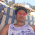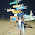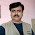## Objective Type Questions on Basic Electrical Engineering -Part16

  A 240V, 60W lamp has a working resistance of:
(a) 1400Ω
(b) 60Ω
(c) 960Ω
(d) 325Ω

 The symbol for the unit of temperature coefficient of resistance is:
(a) Ω /°C
(b)Ω
(c) °C
(d) Ω /Ω°C

 The length of a certain conductor of resistance 100Ω is doubled and its cross-sectional area is halved. Its new resistance is:
(a) 100Ω
(b) 200Ω
(c) 50Ω
(d) 400Ω

 Voltage drop is the:
(a) Maximum potential
(b) Difference in potential between two points
(c) Voltage produced by a source
(d) Voltage at the end of a circuit

 The largest number of 100W electric light bulbs which can be operated from a 240V supply fitted with a 13A fuse is:
(a) 2
(b) 7
(c) 31
(d) 18

 When an atom loses an electron, the atom:
(a) Becomes positively charged
(b) Disintegrates
(c) Experiences no effect at all
(d) Becomes negatively charged

 The energy used by a 1.5kW heater in 5 minutes is:
(a) 5 J
(b) 450 J
(c) 7500 J
(d) 450 000 J

 The unit of resistivity is:
(a) ohms
(b) ohm millimetre
(c) ohm metre
(d) ohm/metre

 A resistor marked as4K7G indicates a value of:
(a) 47Ω ±20%
(b) 4.7Ω k ±20%
(c) 0.47Ω ±10%
(d) 4.7 kΩ ±2

 The resistance of a 2 km length of cable of cross-sectional area 2mm2 and resistivity of 2×10-8Ω m is:
(a) 0.02Ω
(b) 20Ω
(c) 0.02mΩ
(d) 200Ω

 A piece of graphite has a cross-sectional area of 10mm2. If its resistance is 0.1Ω and its resistivity 10×108 Ωm, its length is:
(a) 10 km
(b) 10 cm
(c) 10 mm
(d) 10 m

 A nickel coil has a resistance of 13Ω at 50°C. If the temperature coefficient of resistance at 0°C is 0.006/ °C, the resistance at 0°C is:
(a) 16.9Ω
(b) 10Ω
(c) 43.3Ω
(d) 0.1Ω

 A coil of wire has a resistance of 10 at 0°C. If the temperature coefficient of resistance for the wire is 0.004/ °C, its resistance at 100°C is:
(a) 0.4Ω
(b) 1.4Ω
(c) 14Ω
(d) 10Ω

 A colour coding of red-violet-black on a resistor indicates a value of:
(a) 27Ω ±20%
(b) 270Ω
(c) 270Ω ±20%
(d) 27Ω ±10%

 An inductance of 10mH connected across a 100V, 50 Hz supply has an inductive reactance of
(a) 10π
(b) 1000π
(c) π
(d) π H
9:38 AM

#### 9 comments:

1.ans of Q15 is A

2.Queation no. 10 answer i thiNk wrong it should be 0.02 ohm

1.answer 10 is right

3.Q 10 and Q 15 both are ri8.

4.Some questions are wrong answers ...need improvement...

5.14 correct answer option A

6.7.Very useful topic

8.